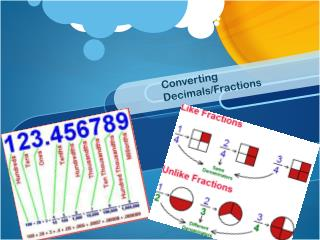DownloadDownload PresentationConverting Decimals/Fractions

# Converting Decimals/Fractions

Télécharger la présentation## Converting Decimals/Fractions

- - - - - - - - - - - - - - - - - - - - - - - - - - - E N D - - - - - - - - - - - - - - - - - - - - - - - - - - -
##### Presentation Transcript

1. Converting Decimals/Fractions

2. Essential Question (EQ): Explain the two steps needed to convert from a decimal number to a fraction. Explain the process of converting a fraction to a decimal. Reducing to Lowest Terms There are two different ways to reduce a fraction to lowest terms. 1. Keep _______ the __________ (top) & ___________ (bottom) by the common _________ until they don’t have anything else in common. 84/100 example on board denominator dividing numerator factors

3. Essential Question (EQ): Explain the two steps needed to convert from a decimal number to a fraction. Explain the process of converting a fraction to a decimal. Reducing to Lowest Terms There are two different ways to reduce a fraction to lowest terms. 2. Known as the ________ method. ladder

4. Essential Question (EQ): Explain the two steps needed to convert from a decimal number to a fraction. Explain the process of converting a fraction to a decimal. How can we use the Ladder method to reduce 24/60? 2 24 60 Divide out all the common factors down the left side. What number goes into both 24 and 60? Does any # besides 1 go into both 12 and 30? 2 12 30 How about 6 and 15? Anything else? 3 6 15 No? You’re done. 2 5

5. The numbers on the bottom of the ladder are your simplified numerator and denominator. Essential Question (EQ): Explain the two steps needed to convert from a decimal number to a fraction. Explain the process of converting a fraction to a decimal. Which numbers in the ladder give you the simplified fraction? # # # # # # # # # # #

6. Essential Question (EQ): Explain the two steps needed to convert from a decimal number to a fraction. Explain the process of converting a fraction to a decimal. How can we use the Ladder method to reduce 24/60? 2 24 60 2 12 30 2 3 6 15 5 2 5

7. Essential Question (EQ): Explain the two steps needed to convert from a decimal number to a fraction. Explain the process of converting a fraction to a decimal. Use the Ladder to reduce 36/60… On the board

8. Essential Question (EQ): Explain the two steps needed to convert from a decimal number to a fraction. Explain the process of converting a fraction to a decimal. Vocabulary –Shoot For The Stars 30 seconds • I got this! I know this word AND I could even teach it to somebody else. • I think I know this word, but I need more practice. • I am clueless! I have no idea what this word means.

9. Essential Question (EQ): Explain the two steps needed to convert from a decimal number to a fraction. Explain the process of converting a fraction to a decimal. Vocabulary – Abbreviate when you can. The number on top of a fraction bar Numerator: __________________________________________ Denominator: ____________________________________________ INDEED! Place Value: ____________________________________________ The number on bottom of a fraction bar The value that a digit has by virtue of its position a the #.

10. Essential Question (EQ): Explain the two steps needed to convert from a decimal number to a fraction. Explain the process of converting a fraction to a decimal. Place Value Example

11. Essential Question (EQ): Explain the two steps needed to convert from a decimal number to a fraction. Explain the process of converting a fraction to a decimal. Commercial Break • Quote of the Day: • “To succeed, you need to find something to hold on to, something to motivate you, something to inspire you.” – Tony Dorsett

12. Essential Question (EQ): Explain the two steps needed to convert from a decimal number to a fraction. Explain the process of converting a fraction to a decimal. THINK/PAIR/SHAREQuickwrite IN YOUR OWN WORDS, WRITE A COMPLETE SENTENCE THAT DESCRIBES AN EASY WAY TO REMEMBER THE DIFFERENCE BETWEEN THE NUMERATOR AND DENOMINATOR.

13. Essential Question (EQ): Explain the two steps needed to convert from a decimal number to a fraction. Explain the process of converting a fraction to a decimal. Fraction to Decimal fraction decimal numerator • To convert a ________ to a __________, I need to divide the __________ by the _____________. In other words, I need to divide the _______ by the _________. 1/2 3/4 3/8 On board denominator top bottom

14. Essential Question (EQ): Explain the two steps needed to convert from a decimal number to a fraction. Explain the process of converting a fraction to a decimal. Decimal to Fraction decimal fraction “Say” • To convert a ________ to a __________, I need to __________ it properly in my head using the __________ __________. Then I just ____________ the ___________ I just said. .25 .7 3.55 On board Place Value reduce fraction

15. Essential Question (EQ): Explain the two steps needed to convert from a decimal number to a fraction. Explain the process of converting a fraction to a decimal. Modeling – Thinking Map CONVERTING Dec to Frac Frac to Dec Top ÷ Bottom Numerator Denominator Say it properly using place values Reduce using Ladder or Other method